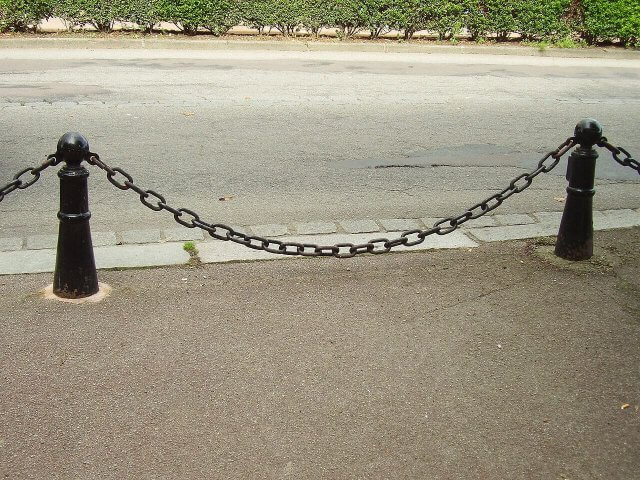# Polygonal Wheel

## Polygon Wheel

Should the wheels always be round?
All polygon wheels can roll smoothly. To do this, you need a suitable floor.

## How is the floor for the polygonal wheel to roll smoothly?

The floor shown in the above simulation is an inverted ‘Catenary.’ Catenaries look similar to ellipses, parabolas, and trigonometric functions but are slightly different.
Assuming a line with a constant line density, if you hold both ends of the line, the rope’s shape hanging down becomes a catenary line.A catenary can be expressed as an exponential function of e (the natural logarithm).

\begin{align} y &= \frac{a}{2}(e^{\frac{x}{a}} + e^{-\frac{x}{a}}) \\&= a \; cosh(\frac{x}{a}) \end{align}

If you set the $$a$$ value to ‘1’, you can get a catenary line like the one below.If you flip the graph about the x-axis, it becomes a catenary line.
Due to the nature of the exponential function of e, all catenary lines resemble each other in shape.